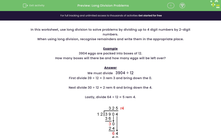# Solve Problems Using Long Division

In this worksheet, students will solve problems involving dividing numbers up to four digits by a two-digit number using the formal written method of long division. There will be remainders, which should be interpreted correctly.Key stage:  KS 2

Curriculum topic:   Number: Addition, Subtraction, Multiplication and Division

Curriculum subtopic:   Divide to Four Digits (Long Division)

Difficulty level:#### Worksheet Overview

In this activity, we will use long division to solve problems by dividing up to four digit numbers by two-digit numbers.

When using long division, we need to recognise remainders and write them in the appropriate place.Example

3,904 eggs are packed into boxes of 12.

How many boxes will there be and how many eggs will be left over?

We must do   3904 ÷ 12

First, do  39 ÷ 12 = 3 rem 3 and bring down the 0.

Next, do 30 ÷ 12 = 2 rem 6 and bring down the 4.

Lastly, divide 64 ÷ 12 = 5 rem 4.We have shown that 3,904 ÷ 12 = 325 r4

There will be 325 boxes and 4 eggs will be left over.

Shall we have a go at some questions now?### What is EdPlace?

We're your National Curriculum aligned online education content provider helping each child succeed in English, maths and science from year 1 to GCSE. With an EdPlace account you’ll be able to track and measure progress, helping each child achieve their best. We build confidence and attainment by personalising each child’s learning at a level that suits them.

Get started